0000

##### 一、选择题（每题8.00分） 亲爱的同学，本部分为选择题，请选择你认为正确的选项。注意，该部分都是单项选择哦！答完选择题之后可以进入到简答题测评。
01 .在数1，0，-1，-2中，最大的数是(     )

A.-2
B.-1
C.0
D.1

02 .实数a、b在数轴上的位置如图所示，则化简代数式|a+b|-a的结果是(         )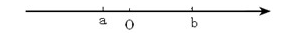A.2a+b
B.2a
C.a

03 .A.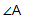B.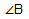C.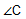D.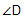04 .用四舍五入法按要求对0.05019分别取近似值，其中错误的是(     )

A.0.1（精确到0.1）
B.0.05（精确到百分位）
C.0.05（精确到千分位）
D.0.0502（精确到0.0001）

05 .下列计算正确的一个是(     )

A.a5+a5=2a10
B.a5+a5=a10
C.a5+a5=2a5
D.x2y+xy2=2x3y3

06 .方程(m+1)x2+3x-5=3 是关于x的一元一次方程，则m的值是(     )

A.m=0
B.m=1
C.m=-1
D.无解

07 .设a为最小的正整数，b是最大的负整数，c是绝对值最小的数，d是倒数等于自身的有理数，则a﹣b+c﹣d的值为(     )

A.1
B.3
C.1或3
D.2或﹣1

08 .如图所示，直线AB，CD相交于点O，OE⊥AB于点O，OF平分∠AOE，∠1=15°30′，则下列结论中不正确的是(     )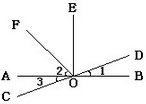A.∠2=45°
B.∠1=∠3
C.∠AOD与∠1互为补角
D.∠1的余角等于75°30′

09 .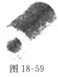A.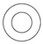B.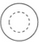C.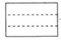D.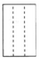10 .下列说法正确的是(      )

A.单项式a的指数为0
B.单项式a的系数为0
C.D.-1是单项式